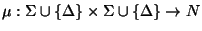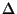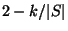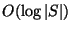Next: MAXIMUM STRING FOLDING Up: Miscellaneous Previous: NEAREST STRING   Index

### MINIMUM TREE ALIGNMENT

• INSTANCE: Set S of sequences over an alphabet, a score schemesatisfying the triangle inequality, and a tree T of bounded degree whose leafs are labeled with the sequences S.

• SOLUTION: A sequence labeling of the interior nodes of the tree T.

• MEASURE: The total alignment cost of the labeled tree, i.e., the sum over all edges (x,y) in the tree of the edit distance d(x,y) between the labels of the endpoints of the edge. The edit distance is the minimum alignment costover all possible alignments x' and y' of x and y. An alignment is obtained by inserting spaces (denoted by) into the original sequence, either between two characters or in the beginning or at the end. x' and y' must have the same length.

• Good News: Admits a PTAS .

• Comment: Variation in which the tree is not given as an input and the objective is to find the tree with the minimum total alignment cost is called MINIMUM GENERALIZED TREE ALIGNMENT or MINIMUM EVOLUTIONARY TREE and is APX-hard . The variation of this latter problem which makes use of the quartet paradigm belongs to PTAS .

MINIMUM SEQUENCE ALIGNMENT, the variation in which the objective is to minimize the sum of edit distances of all pairs of sequences in the alignment, is approximable withinfor any fixed constant. The generalization of MINIMUM SEQUENCE ALIGNMENT to weighted sums is approximable within.Next: MAXIMUM STRING FOLDING Up: Miscellaneous Previous: NEAREST STRING   Index
Viggo Kann
2000-03-20